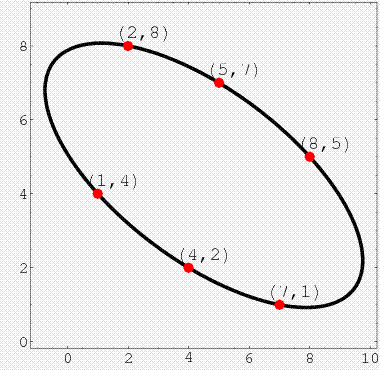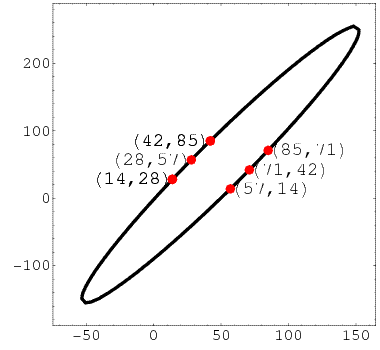# Thread: Cyclic number 142857 and Enneagram

1. 1/7 = 0.(142857)
2/7 = 0.(285714)
3/7 = 0.(428571)
4/7 = 0.(571428)
5/7 = 0.(714285)
6/7 = 0.(857142)

This series of numbers has strange properties
Above, it seems these numbers follow a continuous loop
A better way to visualize this is the "fourth way enneagram"

124578.jpg9 points for the 9 numerals (except 0) you can use to write any real number.

Looking at the values of the fractions, one can trace all 6 the same direction in this closed path.
I find this very interesting, what's the significance of this? Are there other patterns that make such loops?2.

3. I have something better. They make ellipses:4.Originally Posted by Wild CobraI have something better. They make ellipses.
That is pretty cool.
Using that first image, I made two congruent triangles.

coord-cyclic.jpg
Not as good as the ellipses, but it relates.

So, I'm assuming the points were plotted from every 2 digits in each sequence.
The sequences from 1/7, 2/7 and 4/7 corresponded to the red triangle.
The sequences from 3/7, 5/7 and 6/7 correspond to the blue triangle.

These two triangles are congruent.
Approximate side lengths: 3.6, 6.3 and 4.1
Approximate area of figure: 6.8 squares5. It was this number 142857 that got me introduced to The Science Forum yesterday. It just popped into my mind.
I knew about it from way back in the 1960s. I got it from my favourite number 7 by simply doubling and limiting to 2 digits.
Thus 14 28 56, then the 1 of the next double 128 gets added to the 6 to make 142857. Multiply by original 7 and get 999999, so must be reciprocal of 7, if repeated.
I also knew it was very quick to get the other 'sevenths' (2\7, etc.) by just starting the sequence at a different point.
I also knew then about the enneagram and could construct it by drawing a circle, dividing the circumference into 9 parts
(goes exactly 40° - thanks to those ancient Babylonians!). Then number 1 to 9 every 40° and join the points using 142857.
The other three digits 3, 6, 9 - join them with a triangle to get a nice logo!.6.Originally Posted by Elterish[What you said]
It also loves the number 9:

1+4+2+8+5+7 = 27 (9 x 3)
27 huh? 2+7 = 9

14+28+57 = 99

14+28+5+7 = 54 (9 x 6)
54? 5+4 = 9
And you get the idea

Using addition with these numbers, you will run into so many 9's and multiples of 9.

Nine itself is also a magical number. 9^2 = 81.

1/81 = 0.012345679012345679012345679... (skips 8)

8/81 = 0.098765432098765432098765432... (skips 1)
And there's more but I'm done there.

So, yeah. Everything in math is intertwined and connected in a multitude of ways we'll never fully understand.Bookmarks
 Posting Permissions
 You may not post new threads You may not post replies You may not post attachments You may not edit your posts   BB code is On Smilies are On [IMG] code is On [VIDEO] code is On HTML code is Off Trackbacks are Off Pingbacks are Off Refbacks are On Terms of Use Agreement